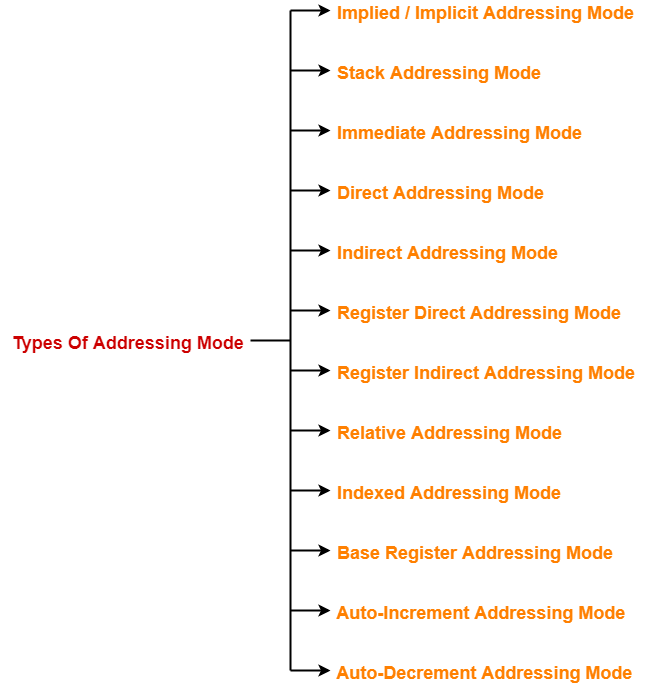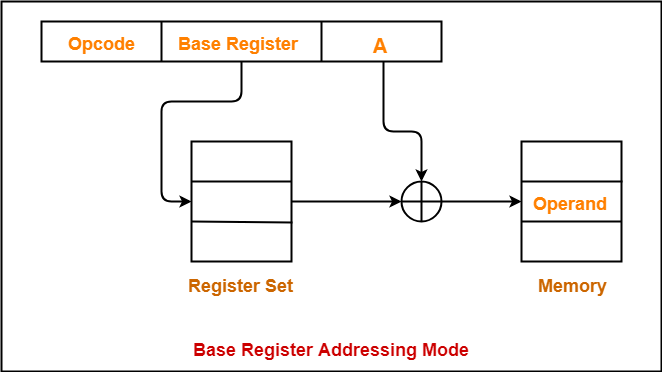# Addressing Modes in Computer Architecture

## Addressing Modes in Computer Architecture-

Before you go through this article, make sure that you have gone through the previous article on Addressing Modes.

We have discussed-

• Addressing modes are the different ways of specifying the location of an operand.
• Various kinds of addressing modes and their applications.In this article, we will discuss syntax used for addressing modes.

## Syntax Of Addressing Modes-

• The computer instructions may use different addressing modes.
• Different addressing modes use different syntax for their representation.

The syntax used for addressing modes are discussed below-

## 1. Immediate Addressing Mode-

# Expression

### Interpretation-

Operand = Expression

### Examples-

• Load R1, #1000 is interpreted as R1 ← 1000
• ADD R2, #3 is interpreted as R2 ← [R2] + 3

## 2. Direct Addressing Mode-

Constant

### Interpretation-

Effective address of the operand = Constant

Operand = Content of the memory location ‘Constant’

Operand = [Constant]

### Examples-

• Load R1, 1000 is interpreted as R1 ← 
• ADD R2, 3 is interpreted as R2 ← [R2] + 

## 3. Register Direct Addressing Mode-

Rn or [Rn]

### Interpretation-

Operand = Content of the register Rn

Operand = [Rn]

### Examples-

• Load R1, R2 is interpreted as R1 ← [R2]
• ADD R1, R2 is interpreted as R1 ← [R1] + [R2]

## 4. Indirect Addressing Mode-

### Syntax-

@Expression or @(Expression) or (Expression)

### Interpretation-

Effective address of the operand = Content of the memory location ‘expression’

Operand = [[ Expression]]

### Examples-

• Load R1, @1000 is interpreted as R1 ← []
• ADD R1, @(1000) is interpreted as R1 ← [R1] + []
• ADD R1, (1000) is interpreted as R1 ← [R1] + []

## 5. Register Indirect Addressing Mode-

### Syntax-

@Rn or @(Rn) or (Rn)

### Interpretation-

Effective address of the operand = Content of the register Rn

Operand = [[ Rn ]]

### Examples-

• Load R1, @R2 is interpreted as R1 ← [[R1]]
• ADD R1, @(R2) is interpreted as R1 ← [R1] + [[R2]]
• ADD R1, (R2) is interpreted as R1 ← [R1] + [[R2]]

## 6. Displacement Addressing Mode-

This addressing mode may be-

• Relative addressing mode
• Index addressing mode
• Base register addressing mode

disp (Rn)

### Interpretation-

Effective address of the operand = disp + Content of the register Rn

Operand = [disp + [ Rn ]]

### Examples-

• Load R1, 100(R2) is interpreted as R1 ← [100 + [R1]]
• ADD R1, 100(R2) is interpreted as R1 ← [R1] + [100 + [R2]]

## 7. Auto-Increment Addressing Mode-

(Rn)+

### Interpretation-

Effective address of the operand = Content of the register Rn

Operand = [[ Rn ]]

After fetching the operand, increment [Rn] by step size ‘d’

### Examples-

• Load R1, (R2)+ is interpreted as R1 ← [[R2]] followed by R2 ← [R2] + d
• ADD R1, (R2)+ is interpreted as R1 ← [R1] + [[R2]] followed by R2 ← [R2] + d

## 8. Auto-Decrement Addressing Mode-

-(Rn)

### Interpretation-

Before fetching the operand, decrement [Rn] by step size ‘d’

Then, Effective address of the operand = Content of the register Rn

Operand = [[ Rn ]]

### Examples-

• Load R1, -(R2) is interpreted as R2 ← [R2] – d followed by R1 ← [[R2]]
• ADD R1, -(R2) is interpreted as R2 ← [R2] – d followed by R1 ← [R1] + [[R2]]

To gain better understanding about Syntax of Addressing Modes,

Watch this Video Lecture

Next Article- Practice Problems On Addressing Modes

Get more notes and other study material of Computer Organization and Architecture.

Watch video lectures by visiting our YouTube channel LearnVidFun.

SummaryArticle Name
Addressing Modes in Computer Architecture
Description
In computer architecture, addressing modes are the different ways of specifying the operand location. Different types of addressing Modes exist. Syntax of addressing mode is the way of representing the addressing mode used.
Author
Publisher Name
Gate Vidyalay
Publisher Logo